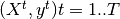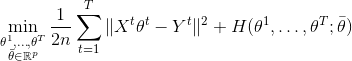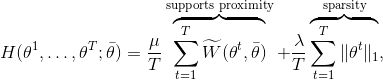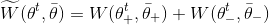Welcome to MTW documentation¶

Wasserestein regularization for sparse Multi-task regression.

Given high dimensional regression datasets, MTW solves the optimization problem:where:withwhere W is the Unbalanced KL Wasserstein distance.

Install the development version¶

From a console or terminal clone the repository and install MTW:

git clone https://github.com/hichamjanati/mtw.git
cd mtw/
conda env create --file environment.yml
source activate mtw-env
pip install --no-deps -e .

Demos & Examples¶

Given a ground metric M and the entropy parameter that define the Wasserstein metric, an MTW object can be created and fitted on multi-task regression data (X, y). Where the shapes of X and Y are (n_tasks, n_samples, n_features) and (n_tasks, n_samples)

>>> from mtw import MTW
>>> import numpy as np
>>> n_tasks, n_samples, n_features = 2, 10, 50
>>> # Compute M as Euclidean distances matrix if not given
>>> grid = np.arange(n_features)
>>> M = (grid[:, None] - grid[None, :]) ** 2
>>> # Some data X and y
>>> X = np.random.randn(n_tasks, n_samples, n_features)
>>> y = np.random.randn(n_tasks, n_samples)
>>> epsilon = 1. / n_features
>>> alpha = 0.1
>>> beta = 0.1
>>> mtw = MTW(alpha=alpha, beta=beta, M=M, epsilon=epsilon)
>>> mtw = mtw.fit(X, y, verbose=False)
>>> coefs = mtw.coefs_

A concomittant version where the standard deviation of each task is inferred. The lower bound on sigma can be set via the sigma0 parameter of MTW. The following example sets this lower bound to 1% of the initial std estimation np.std(Y).

>>> from mtw import MTW
>>> n_tasks, n_samples, n_features = 2, 10, 50
>>> grid = np.arange(n_features)
>>> M = (grid[:, None] - grid[None, :]) ** 2
>>> # Some data X and y
>>> X = np.random.randn(n_tasks, n_samples, n_features)
>>> y = np.random.randn(n_tasks, n_samples)
>>> epsilon = 1. / n_features
>>> alpha = 0.1
>>> beta = 0.1
>>> sigma0 = 0.01  # sigma0 lower bound
>>> mtw = MTW(alpha=alpha, beta=beta, M=M, epsilon=epsilon, sigma0=sigma0)

See ./examples for more.

Dependencies¶

All dependencies are in ./environment.yml

Cite¶

If you use this code, please cite:

@InProceedings{janati19a,
author={Hicham Janati and Marco Cuturi and Alexandre Gramfort},
title={Wasserstein regularization for sparse multi-task regression},
booktitle = {Proceedings of the Twenty-second International Conference on Artificial Intelligence and Statistics},
year =       {2019},
volume =     {89},
series =     {Proceedings of Machine Learning Research},
month =      {16--19 Apr},
publisher =          {PMLR},
}

If you use the concomittant (MWE) version of MTW, please cite:

@InProceedings{janati19b,
author={Hicham Janati and Thomas Bazeille and Bertrand Thirion and Marco Cuturi and Alexandre Gramfort},
title={Group level M-EEG source imaging via Optimal transport: Minimum Wasserstein Estimates},
booktitle = {Proceedings of the Fifty-th Conference on Information Processing and Medical Imaging},
year =       {2019},
month =      {02--07 June},
publisher =          {Springer},
}

API Documentation¶

API Documentation

Examples¶

A set of examples: User Guide.